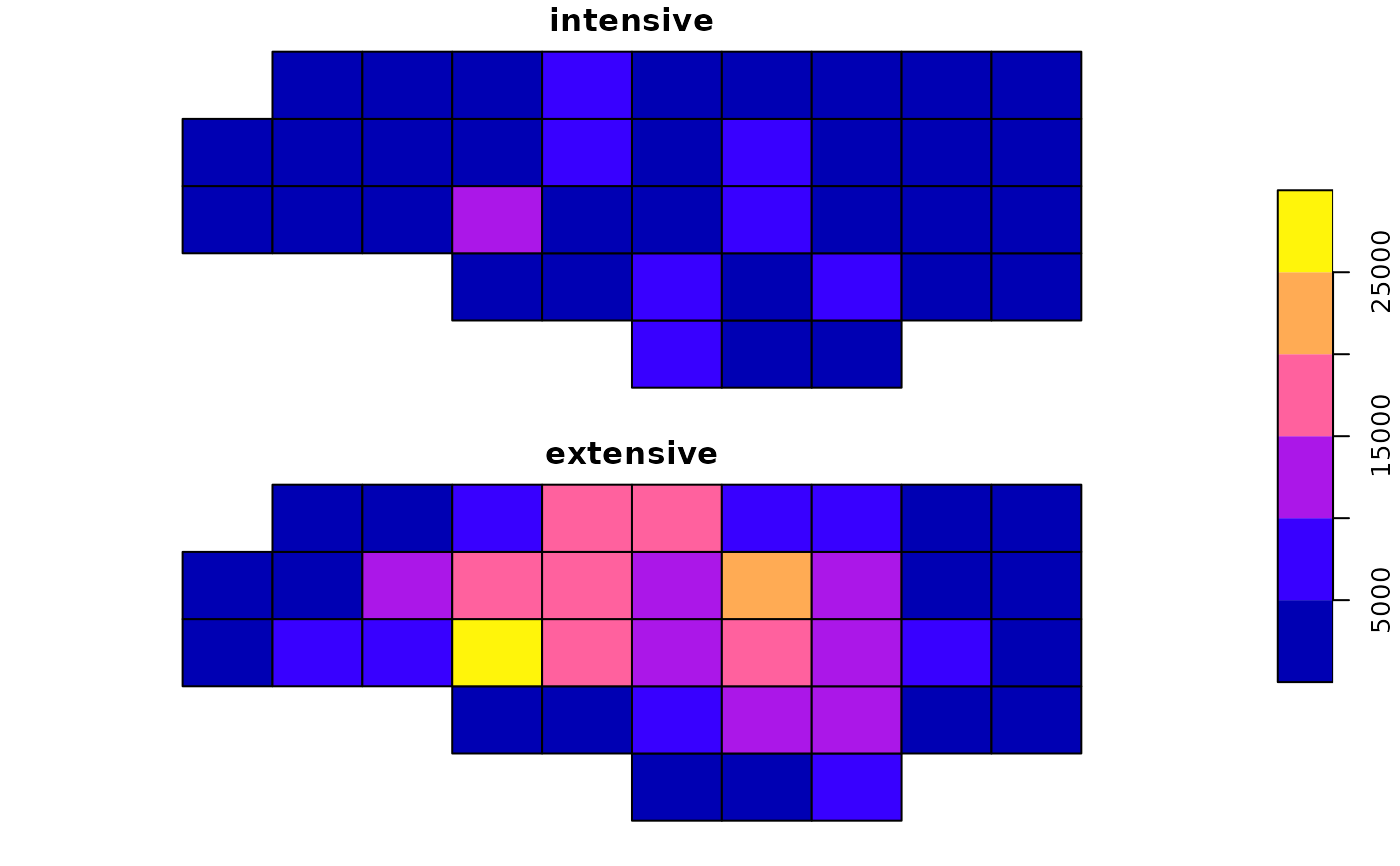Areal-weighted interpolation of polygon data

## Usage

st_interpolate_aw(x, to, extensive, ...)

# S3 method for sf
st_interpolate_aw(x, to, extensive, ..., keep_NA = FALSE)

## Arguments

x

object of class sf, for which we want to aggregate attributes

to

object of class sf or sfc, with the target geometries

extensive

logical; if TRUE, the attribute variables are assumed to be spatially extensive (like population) and the sum is preserved, otherwise, spatially intensive (like population density) and the mean is preserved.

...

ignored

keep_NA

logical; if TRUE, return all features in to, if FALSE return only those with non-NA values (but with row.names the index corresponding to the feature in to)

## Examples

nc = st_read(system.file("shape/nc.shp", package="sf"))
#> Reading layer nc' from data source
#>   /home/runner/work/_temp/Library/sf/shape/nc.shp' using driver ESRI Shapefile'
#> Simple feature collection with 100 features and 14 fields
#> Geometry type: MULTIPOLYGON
#> Dimension:     XY
#> Bounding box:  xmin: -84.32385 ymin: 33.88199 xmax: -75.45698 ymax: 36.58965
g = st_make_grid(nc, n = c(10, 5))
a1 = st_interpolate_aw(nc["BIR74"], g, extensive = FALSE)
#> Warning: st_interpolate_aw assumes attributes are constant or uniform over areas of x
sum(a1$BIR74) / sum(nc$BIR74) # not close to one: property is assumed spatially intensive
#>  0.4026287
a2 = st_interpolate_aw(nc["BIR74"], g, extensive = TRUE)
#> Warning: st_interpolate_aw assumes attributes are constant or uniform over areas of x
# verify mass preservation (pycnophylactic) property:
sum(a2$BIR74) / sum(nc$BIR74)
#>  0.9999998
a1$intensive = a1$BIR74
a1$extensive = a2$BIR74
plot(a1[c("intensive", "extensive")], key.pos = 4)`